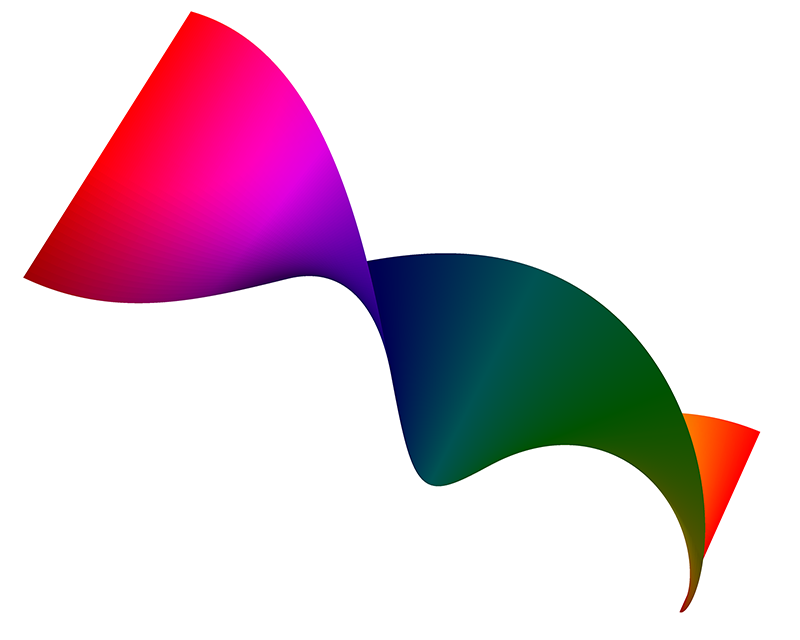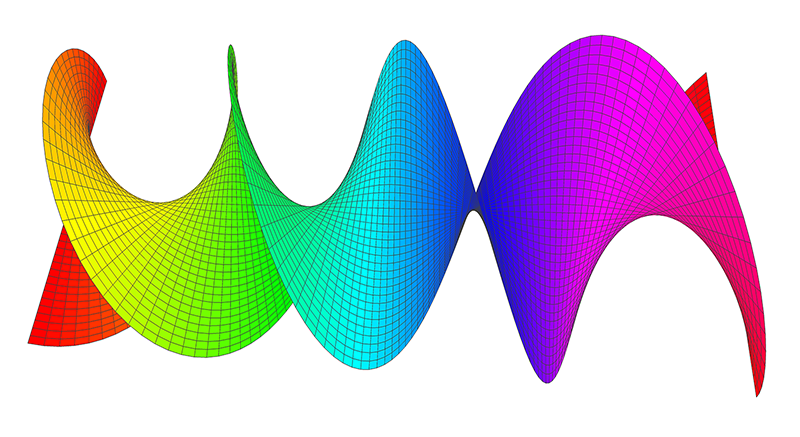# Helicoid Minimal Surface

Written by Paul Bourke
December 2000
x = c v cos(u)
y = c v sin(u)
z = u

-infinity <= u <= infinity
-infinity <= v <= infinity

The helicoid was first documented by J. Meusnier around 1775, it was the first unbounded non-periodic minimal surface to be found. In addition, besides the plane it is the only known ruled minimal surface (zero mean curvature).